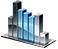Loop Control Statements

# Loop Control Statements

## Break statement

The ` break ` statement is used for termination of the loop (as well as `switch`). After the condition is met, the execution of the nearest outer `while`, `for` or `switch` statement stops.

Example:

` int a=0; uint i=0; array<int>b(5,0); b=1; while(i<b.length()) { System.Print("i="+i); if(b[i]==1)break; i++; } `

Output:

` i=0 i=1 i=2 i=3 `

## Continue statement

The ` continue ` statement is used for skipping the remainder of its body. It transfers control to the beginning of the nearest outer loop, causing the next iteration to start.

Example:

` int a=0; while(a<10) { a++; if(a%2==0) continue; System.Print("a="+a); } `

Output:

` a=1 a=3 a=5 a=7 a=9 `